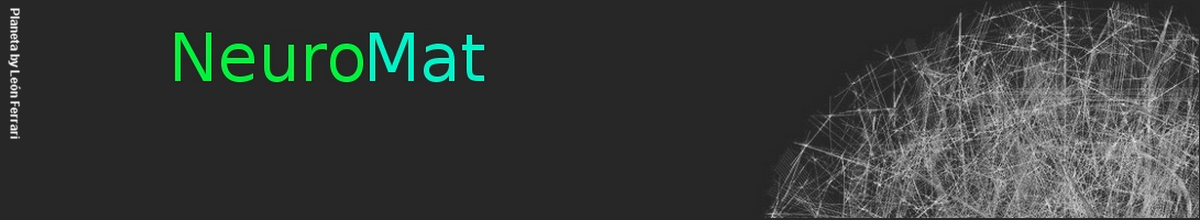### Mean field limits for nonlinear spatially extended Hawkes processes with exponential memory kernels

J. Chevallier, A. Duarte, E. Löcherbach and G. Ost

We consider spatially extended systems of interacting nonlinear Hawkes processes modeling large systems of neurons placed in and study the associated mean field limits. As the total number of neurons tends to infinity, we prove that the evolution of a typical neuron, attached to a given spatial position, can be described by a nonlinear limit differential equation driven by a Poisson random measure. The limit process is described by a neural field equation. As a consequence, we provide a rigorous derivation of the neural field equation based on a thorough mean field analysis.

The whole paper is available here.

NeuroCineMat
Featuring this week: